Examples

Chapter 14 Class 11 Probability
Serial order wise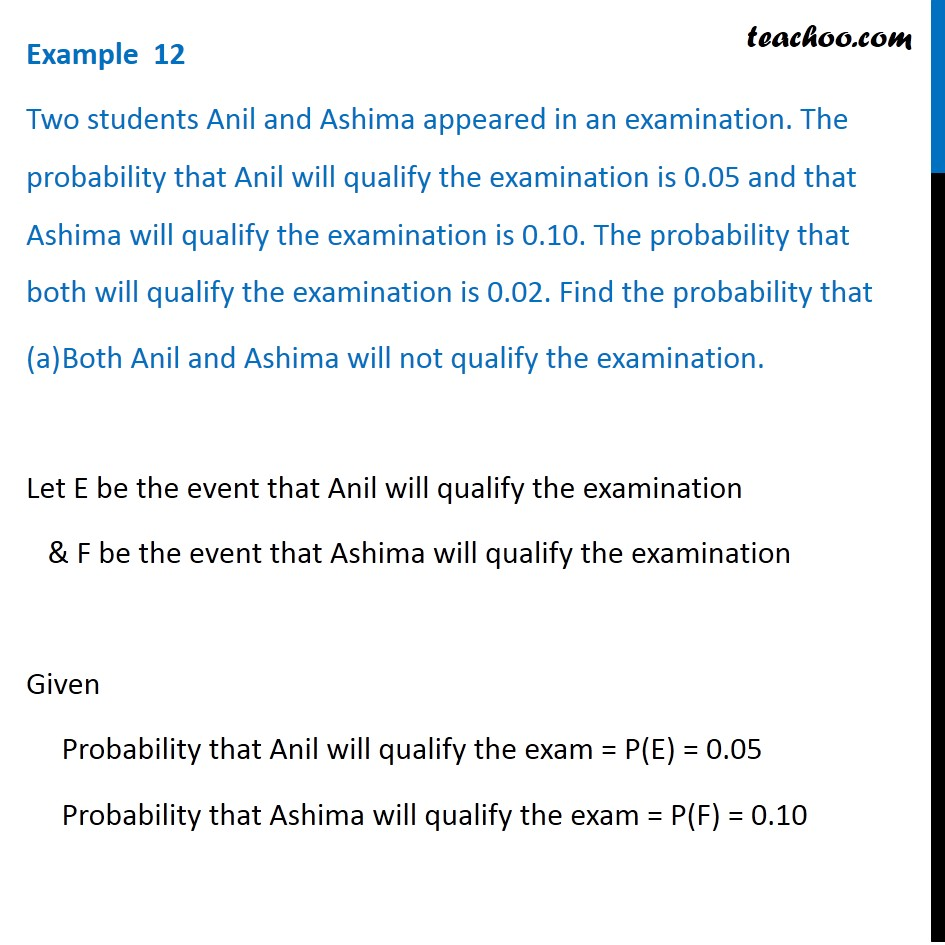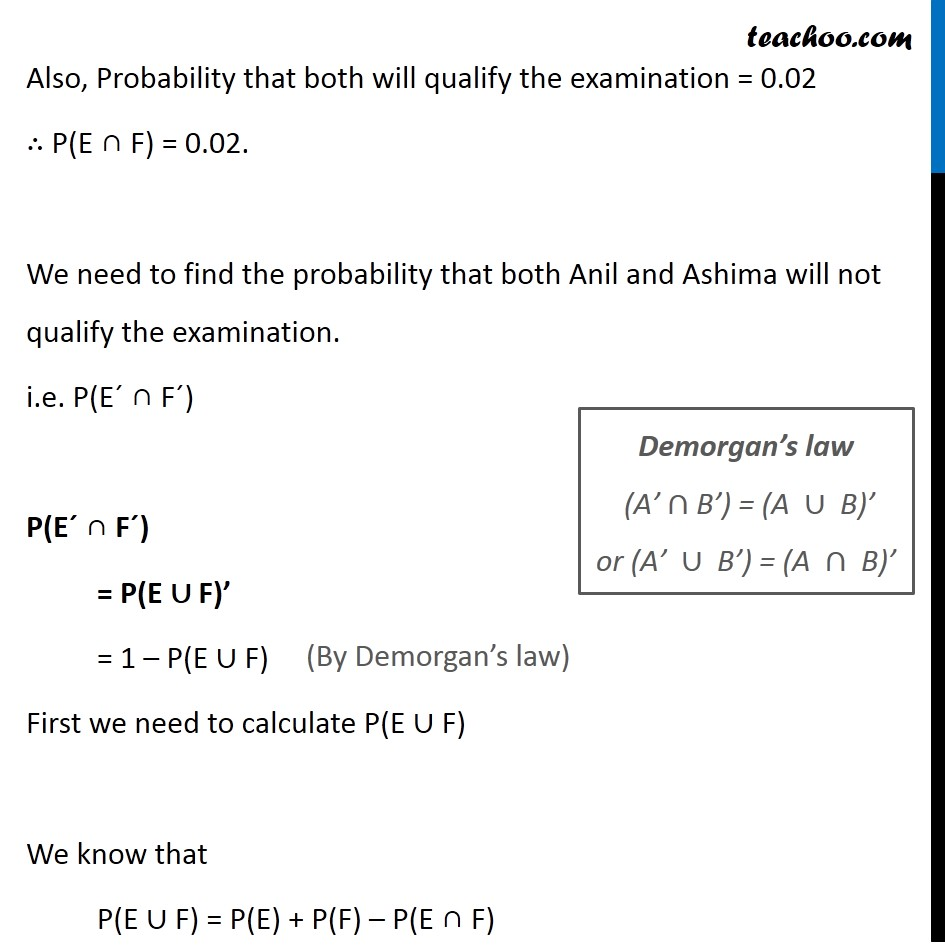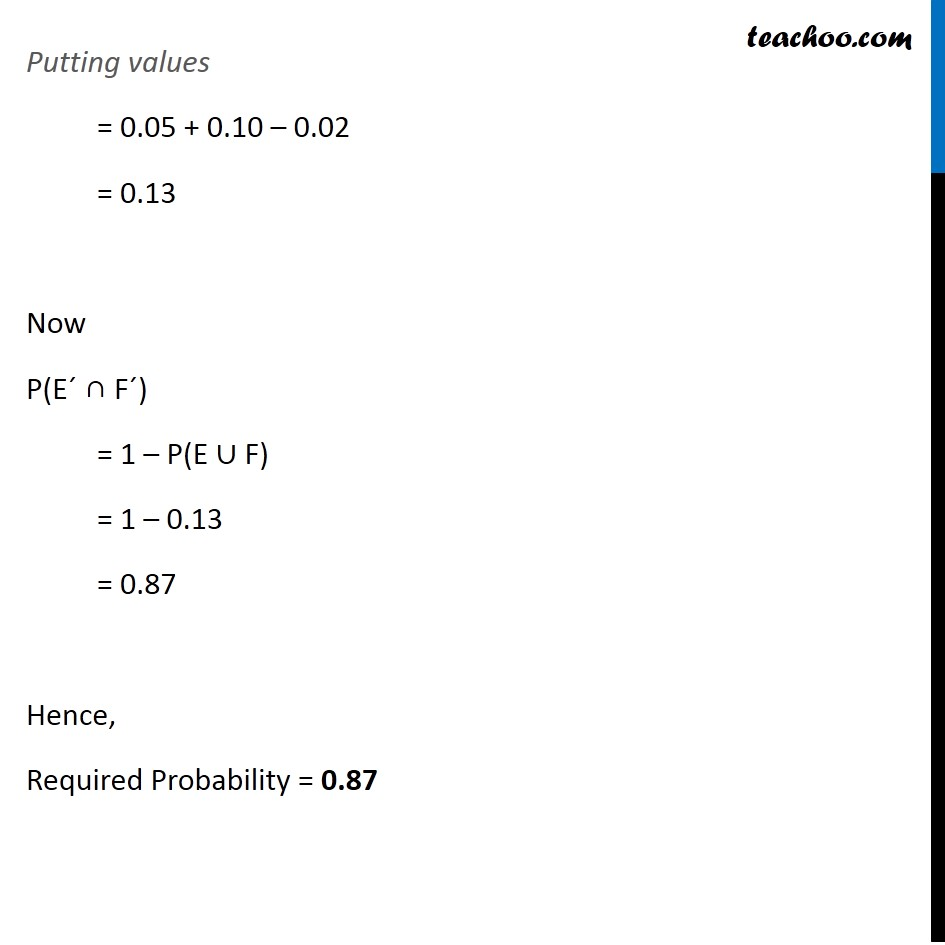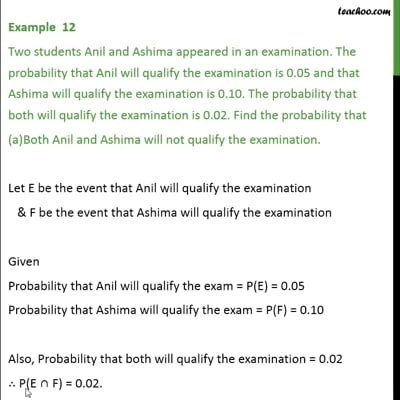This video is only available for Teachoo black users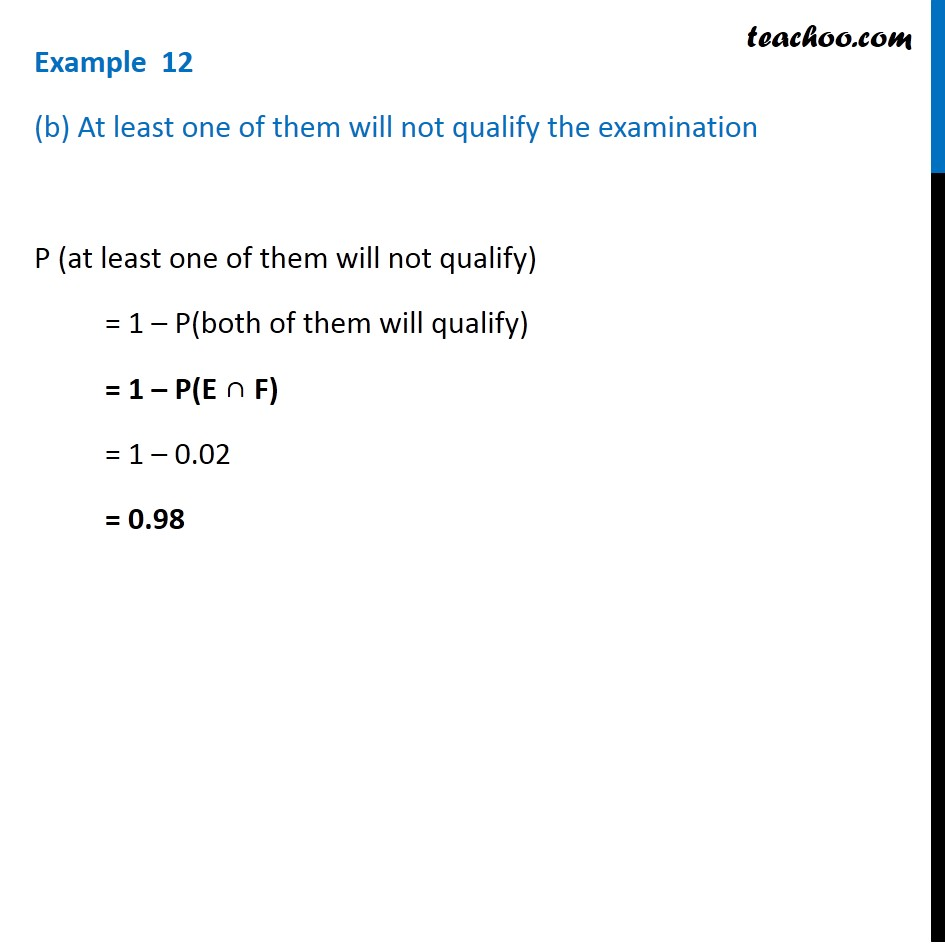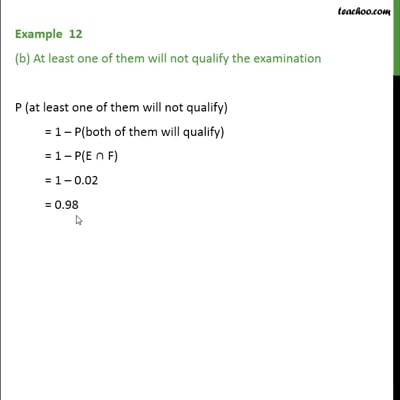This video is only available for Teachoo black users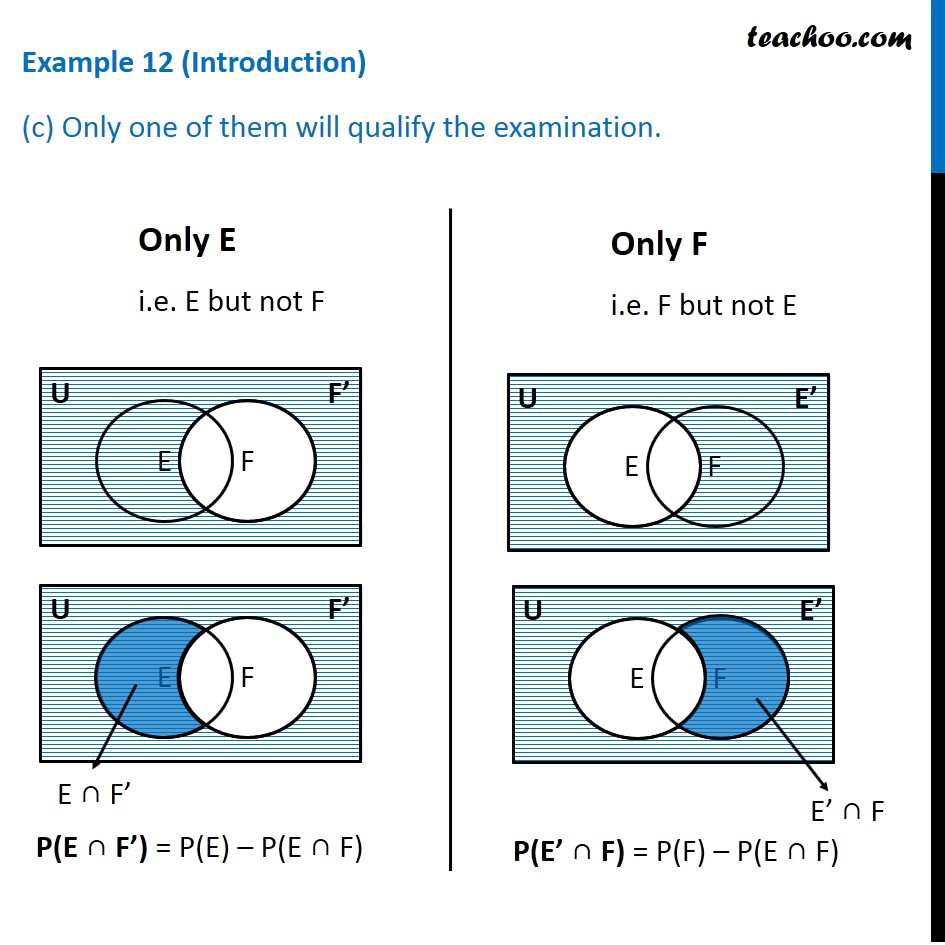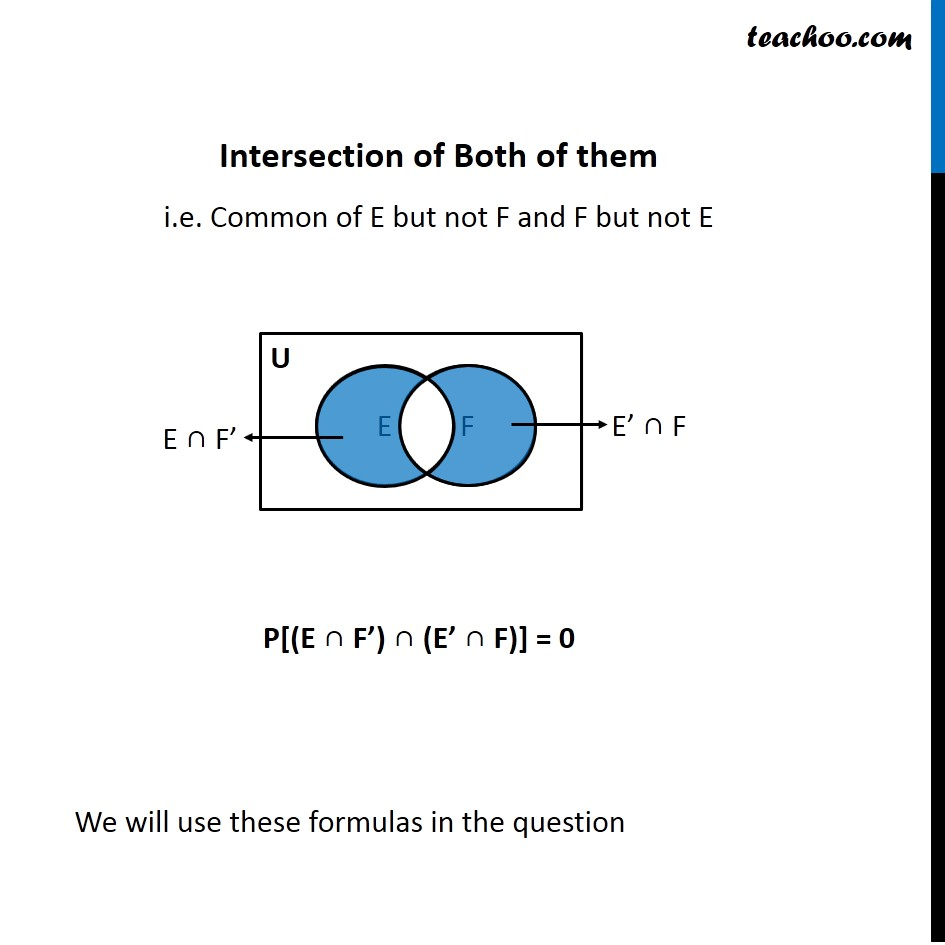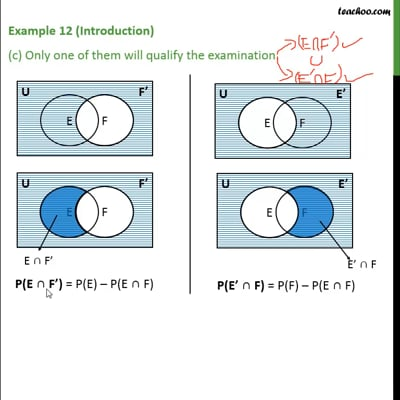This video is only available for Teachoo black users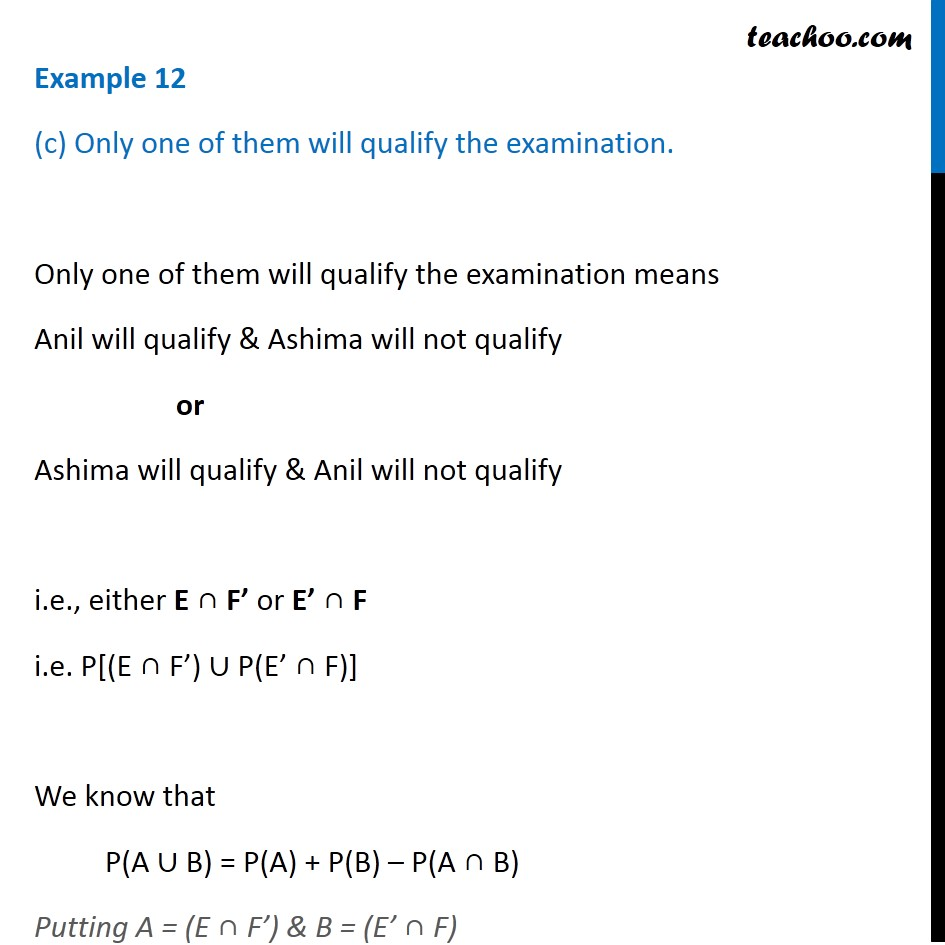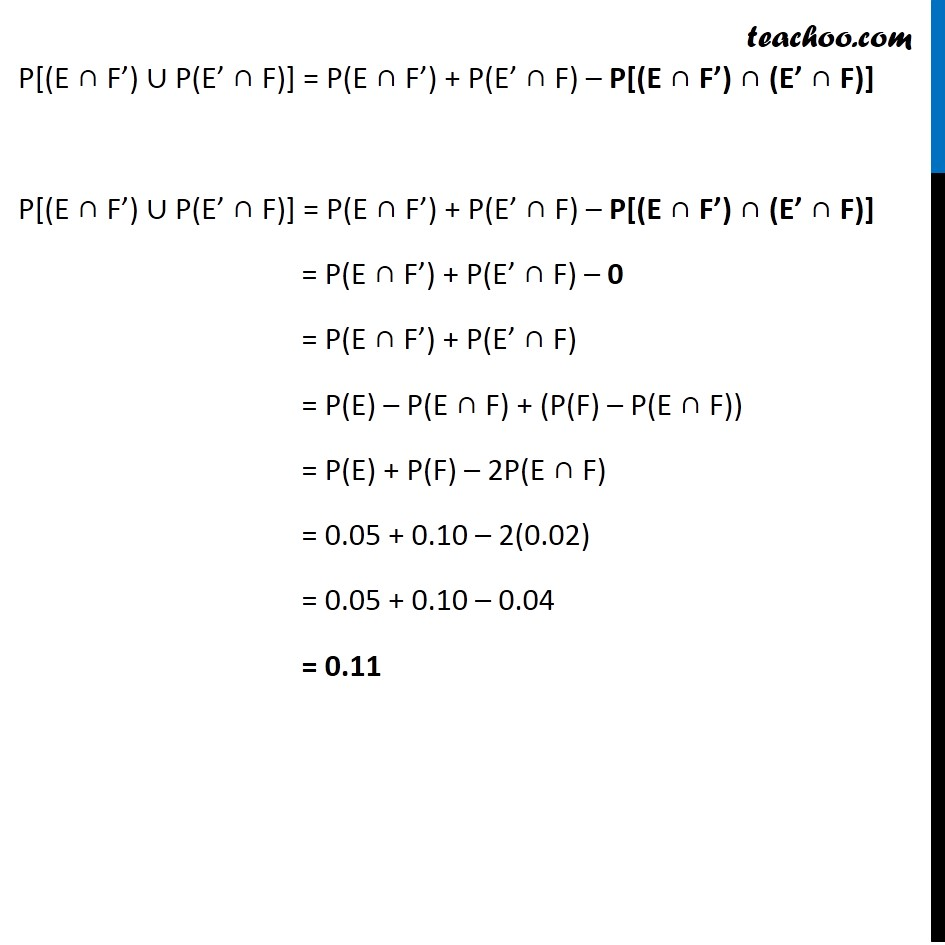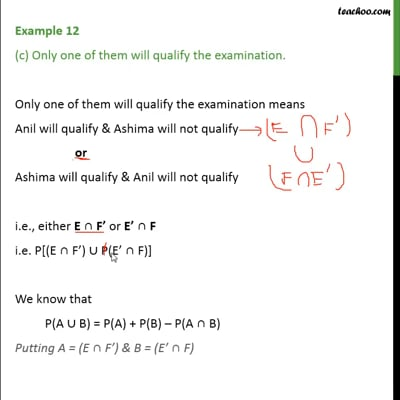This video is only available for Teachoo black users

Learn in your speed, with individual attention - Teachoo Maths 1-on-1 Class

### Transcript

Example 7 Two students Anil and Ashima appeared in an examination. The probability that Anil will qualify the examination is 0.05 and that Ashima will qualify the examination is 0.10. The probability that both will qualify the examination is 0.02. Find the probability that Both Anil and Ashima will not qualify the examination. Let E be the event that Anil will qualify the examination & F be the event that Ashima will qualify the examination Given Probability that Anil will qualify the exam = P(E) = 0.05 Probability that Ashima will qualify the exam = P(F) = 0.10 Also, Probability that both will qualify the examination = 0.02 ∴ P(E ∩ F) = 0.02. We need to find the probability that both Anil and Ashima will not qualify the examination. i.e. P(E´ ∩ F´) P(E´ ∩ F´) = P(E ∪ F)’ = 1 – P(E ∪ F) First we need to calculate P(E ∪ F) We know that P(E ∪ F) = P(E) + P(F) – P(E ∩ F) Demorgan’s law "(A’"∩"B’) = (A "∪" B)’" "or (A’ "∪" B’) = (A "∩" B)’" Putting values = 0.05 + 0.10 – 0.02 = 0.13 Now P(E´ ∩ F´) = 1 – P(E ∪ F) = 1 – 0.13 = 0.87 Hence, Required Probability = 0.87 Example 7 (b) At least one of them will not qualify the examination P (at least one of them will not qualify) = 1 – P(both of them will qualify) = 1 – P(E ∩ F) = 1 – 0.02 = 0.98 Example 12 (Introduction) (c) Only one of them will qualify the examination. P(E ∩ F’) = P(E) – P(E ∩ F) P(E’ ∩ F) = P(F) – P(E ∩ F) Intersection of Both of them i.e. Common of E but not F and F but not E P[(E ∩ F’) ∩ (E’ ∩ F)] = 0 We will use these formulas in the question Example 12 (c) Only one of them will qualify the examination. Only one of them will qualify the examination means Anil will qualify & Ashima will not qualify or Ashima will qualify & Anil will not qualify i.e., either E ∩ F’ or E’ ∩ F i.e. P[(E ∩ F’) ∪ P(E’ ∩ F)] We know that P(A ∪ B) = P(A) + P(B) – P(A ∩ B) Putting A = (E ∩ F’) & B = (E’ ∩ F) P[(E ∩ F’) ∪ P(E’ ∩ F)] = P(E ∩ F’) + P(E’ ∩ F) – P[(E ∩ F’) ∩ (E’ ∩ F)] P[(E ∩ F’) ∪ P(E’ ∩ F)] = P(E ∩ F’) + P(E’ ∩ F) – P[(E ∩ F’) ∩ (E’ ∩ F)] = P(E ∩ F’) + P(E’ ∩ F) – 0 = P(E ∩ F’) + P(E’ ∩ F) = P(E) – P(E ∩ F) + (P(F) – P(E ∩ F)) = P(E) + P(F) – 2P(E ∩ F) = 0.05 + 0.10 – 2(0.02) = 0.05 + 0.10 – 0.04 = 0.11